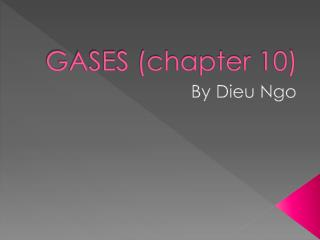DownloadDownload PresentationGASES (chapter 10)

# GASES (chapter 10)

Download Presentation## GASES (chapter 10)

- - - - - - - - - - - - - - - - - - - - - - - - - - - E N D - - - - - - - - - - - - - - - - - - - - - - - - - - -
##### Presentation Transcript

1. GASES (chapter 10) By Dieu Ngo

2. Characteristics of Gases • Essential for life • Expand to fill container • Compressible • Readily flow • Form homogeneous mixtures with other gases • Vol of gases itself=smaller portion of total volume at room temp. and pressure • Noble gases=monotomic gases • Diatomic gases • Gases: molecular compounds, nonmetals • Vapors

3. Properties of Gases • T- temperature (in K) • V- volume (in L) • n- quantity (in moles) • P- pressure (in atm)

4. Gas Pressure • P=F/A • Barometer-contains a column of mercury (Hg) whose height is directly related to the pressure exerted by the atmosphere (mm Hg or torr) • Manometer- used to measure pressure in enclosed gases (usually below atm. Pressure); det’ed from the difference in heights of mercury levels in U-tube

5. Atmospheric Pressure • Caused by gravity • Atoms of atm.=tiny massesoverride gravitational forces • Magnitude: F= ma • a = 9.8 m/s^2

6. Standard Atmospheric Pressure • PRESSURE= 1 atm = 760 mmHg = 760 torr = 1.013E5Pa = 101.3kPa • Pgas= Patm+Ph

7. Gas Laws • Boyle’s Law- V=contant/P (T, n) ;or P1V1=P2V2 • Charles Law- V= constant x T (P, n); or V1/T1=V2/T2 • Gay-Lussac’s Law- V= constant x n (P, T); or P1/T1=P2/T2

8. Ideal-Gas Equation • PV=nRT • P in atm • V in L • n in moles • T in K • R is gas constant = 0.0821 L-atm/mol-K • Combined: PV/T=PV/T

9. Standard temp. and pressure • STP: • Pressure = 1 atm • Temperature = 0 degrees Celsius = 273K • Volume of 1 mol= 22.4 L

10. Dalton’s Law of Partial Pressure • The total pressure of a mixture of ideal gases is the sum of the individual pressures each ideal gas would exert if it were the only gas in the container • Pt=P1+P2+…+PN • Pi=niRT/V • Pt=Pgas+PH2O

11. Mole Fraction (X) • The ratio of the # of moles of a component in a mixture of the total # of moles present • Xi = #of moles of component i / total # of moles in mixture • PA = (XA)(PT) • Vol % of a gas in a mixture = mole fraction of that gas

12. Kinetic Molecular Theoryof Gases • KMT- at the same temperature, molecules of all gases have the same average translational kinetic energy • rms speed is the square root of the average of the squared velocities of the gas molecules: • The average speed is the average of the velocities: • Temp increases  speed increases • The rms speed of a gas is inversely related to the molar mass of the gas at a given temp:

13. Effusion • Is the flow of gas molecules through a small pinhole or small opening into a vacuum • Graham’s Law of Effusion relates the rates of 2 gases escaping through the same pinhole and their molar masses at constant temperature

14. Lalalalalalala… chemistry! • Diffusion- A process in which a substance gradually mixes with another • Mean Free Path- the average distance gas molecules travel between collisions

15. Gas molecules vs. ideal gas • Gas molecules- attracts at short distances  do not collide with walls of container like ideal gas • Cause the experimental gas pressure to be less than the ideal-gas pressure • Vol for gas to move in container < total vol of container • Van der Waals equation: